How Cheenta works to ensure student success?
Explore the Back-Story

# AMC 8 2018 Problem 24 | American Mathematics CompetitionsThis is a solution to a problem from American Mathematics Competition (AMC) 8 2020 Problem 18 based on Geometry.

## AMC 8 2018 Problem 24

In the cubewith opposite vertices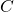and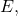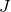and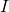are the midpoints of edgesand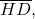respectively. Let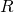be the ratio of the area of the cross-sectionto the area of one of the faces of the cube. What is(A)(B)(C)(D)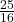(E).

## Solution:

### Useful Resources

This is a solution to a problem from American Mathematics Competition (AMC) 8 2020 Problem 18 based on Geometry.

## AMC 8 2018 Problem 24

In the cubewith opposite verticesandandare the midpoints of edgesandrespectively. Letbe the ratio of the area of the cross-sectionto the area of one of the faces of the cube. What is(A)(B)(C)(D)(E).

## Solution:

### Useful Resources

This site uses Akismet to reduce spam. Learn how your comment data is processed.

### Knowledge Partner